Operations with polynomialsCustom SearchOPERATIONS WITH POLYNOMIALS Adding and subtracting polynomials is simply the adding and subtracting of their like terms. There is a great similarity between the operations with polynomials and denominate numbers. Compare the following examples: 1. Add 5 qt and 1 pt to 3 qt and 2 pt.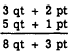2. Add 5x + y to 3x + 2y.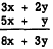One method of adding polynomials (shown in the above examples) is to place like terms in columns and to find the algebraic sum of the like terms. For example, to add 3a + b - 3c, 3b + c - d, and 2a + 4d, we would arrange the polynomials as follows:Subtraction may be performed by using the same arrangement-that is, by placing terms of the subtrahend under the like terms of the minuend and carrying out the subtraction with due regard for sign. Remember, in subtraction the signs of all the terms of the subtrahend must first be mentally changed and then the process completed as in addition. For example, subtract 10a + b from 8a - 2b, as follows:Again, note the similarity between this type of subtraction and the subtraction of denominate numbers. Addition and subtraction of polynomials also can be indicated with the aid of symbols of grouping. The rule regarding changes of sign when removing parentheses preceded by a minus sign automatically takes care of subtraction. For example, to subtract 10a + b from 8a - 2b, we can use the following arrangement: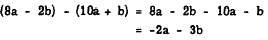Similarly, to add -3x + 2y to -4x - 5y, we can writePractice problems. Add as indicated, in each of the following problems: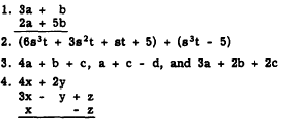In problems 5 through 8, perform the indicated operations and combine like terms. 5. (2a + b) - (3a + 5b) 6. (5x3y + 3x2y) - (x3y) 7. (x + 6) + (3x + 7) 8. (4a2 - b) - (2a2 + b) Answers: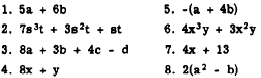MULTIPLICATION OF A POLYNOMIAL BY A MONOMIAL We can explain the multiplication of a polynomial by a monomial by using an arithmetic example. Let it be required to multiply the binomial expression, 7 - 2, by 4. We may write this 4 x (7 - 2)orsimply 4(7 - 2). Now 7 - 2 = 5. Therefore, 4(7 - 2) = 4(5) = 20. Now, let us then subtract. Thus, 4(7 - 2) = (4 x 7) - (4 x 2) = 20. Both methods give the same result. The second method makes use of the distributive law of multiplication. When there are literal parts in the expression to be multiplied, the first method cannot be used and the distributive method must be employed. This is illustrated in the following examples: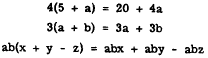Thus, to multiply a polynomial by a monomial, multiply each term of the polynomial by the monomial. Practice problems. Multiply as indicated:Integrated Publishing, Inc. - A (SDVOSB) Service Disabled Veteran Owned Small Business